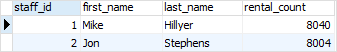# PostgreSQL Tutorial: CTE

August 7, 2023

Summary: in this tutorial, you will learn how to use the PostgreSQL CTE (common table expressions) to simplify complex queries.

## Introduction to PostgreSQL common table expressions or CTEs

A common table expression is a temporary result set which you can reference within another SQL statement including `SELECT`, `INSERT`, `UPDATE` or `DELETE`.

Common Table Expressions are temporary in the sense that they only exist during the execution of the query.

The following shows the syntax of creating a CTE:

``````WITH cte_name (column_list) AS (
CTE_query_definition
)
statement;
``````

In this syntax:

• First, specify the name of the CTE following by an optional column list.
• Second, inside the body of the `WITH` clause, specify a query that returns a result set. If you do not explicitly specify the column list after the CTE name, the select list of the `CTE_query_definition` will become the column list of the CTE.
• Third, use the CTE like a table or view in the `statement` which can be a `SELECT`, `INSERT`, `UPDATE`, or `DELETE`.

Common Table Expressions or CTEs are typically used to simplify complex joins and subqueries in PostgreSQL.

## PostgreSQL CTE examples

Let’s take some examples of using CTEs to get a better understanding.

### A simple PostgreSQL CTE example

We will use the `film` and `rental` tables from the sample database for the demonstration.

See the following example:

``````WITH cte_film AS (
SELECT
film_id,
title,
(CASE
WHEN length < 30 THEN 'Short'
WHEN length < 90 THEN 'Medium'
ELSE 'Long'
END) length
FROM
film
)
SELECT
film_id,
title,
length
FROM
cte_film
WHERE
length = 'Long'
ORDER BY
title;
``````

Here is the partial output:Note that this example is for the demonstration purpose.

In this example, we first defined a common table expression named `cte_film` using the `WITH` clause as follows:

``````WITH cte_film AS (
SELECT
film_id,
title,
(CASE
WHEN length < 30
THEN 'Short'
WHEN length >= 30 AND length < 90
THEN 'Medium'
WHEN length >=  90
THEN 'Long'
END) length
FROM
film
)
``````

The common table expression has two parts:

• The first part defines the name of the CTE which is `cte_film`.
• The second part defines a `SELECT` statement that populates the expression with rows.

We then used the `cte_film` CTE in the `SELECT` statement to return only films whose lengths are ‘Long’.

### Joining a CTE with a table example

In the following example, we will use the `rental` and `staff` tables:

The following statement illustrates how to join a CTE with a table:

``````WITH cte_rental AS (
SELECT staff_id,
COUNT(rental_id) rental_count
FROM   rental
GROUP  BY staff_id
)
SELECT s.staff_id,
first_name,
last_name,
rental_count
FROM staff s
INNER JOIN cte_rental USING (staff_id);
``````

In this example:

• First, the CTE returns a result set that includes staff id and the number of rentals.
• Then, join the `staff` table with the CTE using the `staff_id` column.

Here is the output:### Using CTE with a window function example

The following statement illustrates how to use the CTE with the `RANK()` window function:

``````WITH cte_film AS  (
SELECT film_id,
title,
rating,
length,
RANK() OVER (
PARTITION BY rating
ORDER BY length DESC)
length_rank
FROM
film
)
SELECT *
FROM cte_film
WHERE length_rank = 1;
``````

In this example:

• First, we defined a CTE that returns the film ranking by length for each film rating.
• Second, we selected only films whose length rankings are one.

The following picture shows the output: